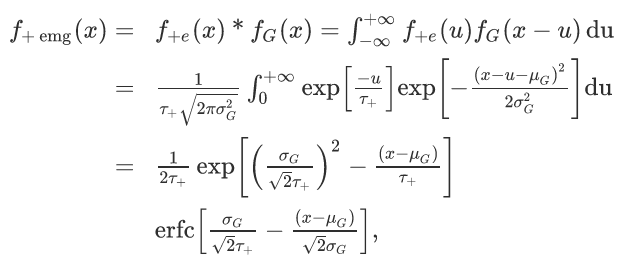# Fit efficieny of long analytical function with many fit parameters

Dear all,

I’ve been using Roofit for a long time now and have never spent too much time optimizing the performance of my fit function. With this post I would like to ask for recommendations and suggestions.

The problem:
Fitting a sum function (“hyperEMG”) of several exponentially-modified Gaussians (“EMG”) to a TH1D (the idea behind the hyperEMG is published here).

The EMG is defined as the convolution of a Gaussian with an exponential distribution, which has an analytical representation in the form of:including the Gaussian mean and variance mu and sigma, and the decay constant tau from the exponential

The hyperEMG is the sum of n EMGs with negative decay constants and m EMGs with positive decay constants. For my data, the Gaussian mean and variance are shared between the different EMGs.

My approach
As I did not want to calculate the convolution, I implemented the analytical expression of the EMG. Each hyperEMG is built from a RooGenericPdf, which takes the analytical function as a string. Consider a hyperEMG with one negative and two positive components. In terms of RooRealVar we have one sigma, one mu, three tau, and two ratios to mix the three EMGs together. As a function string this looks like this

``````@4*1/(2*@3)*exp((@2/(1.4142*@3))^2+(@0-@1)/@3)*TMath::Erfc(@2/(1.4142*@3)+(@0-@1)/(1.4142*@2))+@6*1/(2*@5)*exp((@2/(1.4142*@5))^2-(@0-@1)/@5)*TMath::Erfc(@2/(1.4142*@5)-(@0-@1)/(1.4142*@2))+(1-@4-@6)*1/(2*@7)*exp((@2/(1.4142*@7))^2-(@0-@1)/@7)*TMath::Erfc(@2/(1.4142*@7)-(@0-@1)/(1.4142*@2))
``````

where the RooRealVar are denoted with the @ symbol.

Now, this is for one species in my spectrum, which can consist of many species. The assumption is that all species share the same sigma and tau parameters, as well as the EMG mixing ratios, which means that those RooRealVar are used for all species. Now considering we use the abovementioned function for three different species, we will have to fit three mu, one sigma, three tau, two EMG mixing ratios, and two mixing ratios for the three species, resulting in 11 fit parameters. The mixing of the different species is eventually being done using RooAddPdf on the three individual RooGenericPdf.

This example is the most complex I could fit so far. Having to fit more species or having to use more EMG components results in a very inefficient fitting, even though the fit eventually converges.

The question
Without asking for an explicit implementation of this problem, how would you tackle this (strictly conceptually speaking)?

Finally, here is an example where this three-component hyperEMG is fitted to real data.

Thank you,

Lukas

1 Like

Hello @Lukas.Nies, welcome to the ROOT forum!

This is a very interesting fit! I am looking for some complicated and realistic fits to benchmark and optimize RooFit, would you mind sharing the code for this fit? I don’t need the real data, just the code to create the model so I can generate and fit toy datasets would be enough. This would be a great service for RooFit.

About optimizing your fit: putting everything in one RooGenericPdf. As I explained for example in slide 43 in this talk, the point of RooFit is to have a granular computation graph for your model, so that only few expressions need to be re-evaluated when the minimizer changes one parameter. By putting everything in one RooGenericPdf, the whole expression needs to be re-evaluated if only one parameter is changed. In other words, you are completely bypassing RooFits caching.

The other – probably more important – bottleneck is numeric integration. RooFit assumes that a RooGenericPdf is not necessarily normalized, and it normalizes it with a numeric integral. These numeric integrals are also not cheap. Do you know the analytical integral of your function? Or is it already normalized? In that case it would be better to implement a new RooFit class for this pdf, a `RooEMG`. Then, we can override the methods for analytical integration, and also support vectorized evaluation CPU and evaluation on the GPU, which should definitely fix your performance problems.

You think such a class would be useful? It’s nice that there is also a paper about this distribution, that gives the RooFit implementation more justification.

Cheers,
Jonas

Dear Jonas,

I am happy that this problem may be of interest for the RooFit devs and others from the community.
I have my code on GitHub (please have a look at the dev branch). The example folder has a minimal working example that does not rely on the rest of the repository.

If I run method 1 in the example code (building two hyperEMG(1,2) to represent the two peaks in the data set as RooGenericPdf and then add them with RooAddPdf) I get a very poor run time:

`[#1] INFO:Minization -- Command timer: Real time 3:07:01, CP time 11221.060 [#1] INFO:Minization -- Session timer: Real time 3:14:46, CP time 11686.150, 3 slices`

If I run method 2 (build the sum of the two hyperEMG(1,2) with one single RooGenericPdf), then the run time is much faster:

`[#1] INFO:Minization -- Command timer: Real time 0:00:07, CP time 7.040 [#1] INFO:Minization -- Session timer: Real time 0:00:07, CP time 7.500, 3 slices`

If I build the RooGenericPdf from method 2 and then put it through a RooAddPdf without adding a second pdf, the run time is very slow again. I suppose this has to do with how RooAddPdf treats adding Pdfs? The second method probably does not benefit from the RooFit caching you explained. As far as I can tell the single EMG as defined in the publication is normalized and also gives the analytical integral.

Thanks,

Lukas

This topic was automatically closed 14 days after the last reply. New replies are no longer allowed.